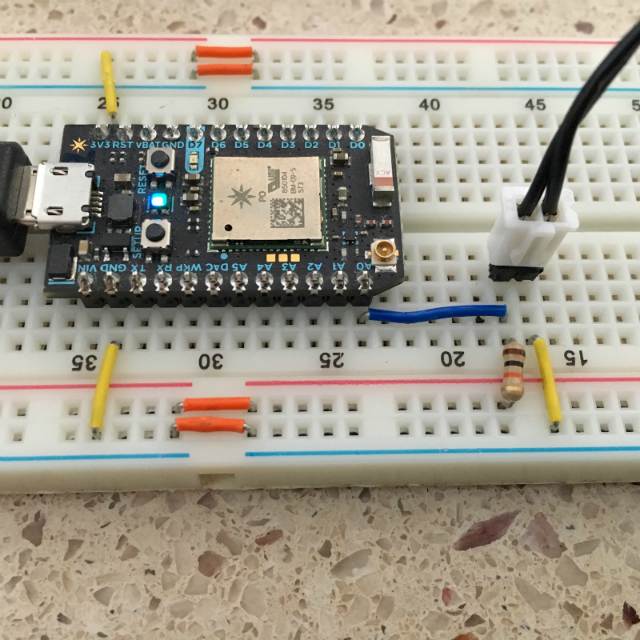# kegnet/photon-thermistor

A thermistor library for Particle Photon
C++
Latest commit d2795c0 Mar 24, 2018
Type Name Latest commit message Commit time
Failed to load latest commit information.examples/temperaturesrc Mar 24, 2018.gitignoreLICENSEREADME.mdbreadboard_hookup.pnglibrary.propertiesverification.txt

# photon-thermistor

A fully configurable thermistor library for Particle Photon and other devices.

Simplified for Particle but also allows for full configuration of Vcc, analog reference voltage, and ADC max. Most thermistor libraries assume ADC max is 1023 but ADC max on Particle is 4095!

For NTC (negative temperature coefficient) thermistors only.

### Hookup### Configuration

Configuration is done via constructor parameters as follows:

Particle constructor, sets defaults: vcc=3.3, analogReference=3.3, adcMax=4095

``````1. pin: Photon analog pin
2. seriesResistor: The ohms value of the fixed resistor (based on your hardware setup, usually 10k)
3. thermistorNominal: Resistance at nominal temperature (will be documented with the thermistor, usually 10k)
4. temperatureNominal: Temperature for nominal resistance in celcius (will be documented with the thermistor, assume 25 if not stated)
5. bCoef: Beta coefficient (or constant) of the thermistor (will be documented with the thermistor, typically 3380, 3435, or 3950)
6. samples: Number of analog samples to average (for smoothing)
7. sampleDelay: Milliseconds between samples (for smoothing)

Thermistor(int pin, int seriesResistor, int thermistorNominal, int temperatureNominal, int bCoef, int samples, int sampleDelay)
``````

General arduino constructor, sets defaults: vcc=3.3, analogReference=3.3

``````1. pin: Photon analog pin
2. seriesResistor: The ohms value of the fixed resistor (based on your hardware setup, usually 10k)
3. adcMax: The maximum analog-to-digital convert value returned by analogRead (Photon is 4095 NOT the typical Arduino 1023!)
4. thermistorNominal: Resistance at nominal temperature (will be documented with the thermistor, usually 10k)
5. temperatureNominal: Temperature for nominal resistance in celcius (will be documented with the thermistor, assume 25 if not stated)
6. bCoef: Beta coefficient (or constant) of the thermistor (will be documented with the thermistor, typically 3380, 3435, or 3950)
7. samples: Number of analog samples to average (for smoothing)
8. sampleDelay: Milliseconds between samples (for smoothing)

Thermistor(int pin, int seriesResistor, int adcMax, int thermistorNominal, int temperatureNominal, int bCoef, int samples, int sampleDelay)
``````

Full constructor, no defaults (useful for ESP8266)

``````1. pin: Photon analog pin
2: vcc: Input voltage (3.3, 5, or something else if you're using a voltage divider)
3: analogReference: reference voltage. Typically the same as vcc, but not always (ie ESP8266=1.0)
4: seriesResistor: The ohms value of the fixed resistor (based on your hardware setup, usually 10k)
5: adcMax: The maximum analog-to-digital convert value returned by analogRead (Photon is 4095 NOT the typical Arduino 1023!)
6: thermistorNominal: Resistance at nominal temperature (will be documented with the thermistor, usually 10k)
7: temperatureNominal: Temperature for nominal resistance in celcius (will be documented with the thermistor, assume 25 if not stated)
8: bCoef: Beta coefficient (or constant) of the thermistor (will be documented with the thermistor, typically 3380, 3435, or 3950)
9: samples: Number of analog samples to average (for smoothing)
10: sampleDelay: Milliseconds between samples (for smoothing)

Thermistor(int pin, float vcc, float analogReference, int seriesResistor, int adcMax, int thermistorNominal, int temperatureNominal, int bCoef, int samples, int sampleDelay)
``````

### Example

``````#include "photon-thermistor.h"

Thermistor *thermistor;

void setup() {
thermistor = new Thermistor(A0, 10000, 10000, 25, 3950, 5, 20);
}

void loop() {